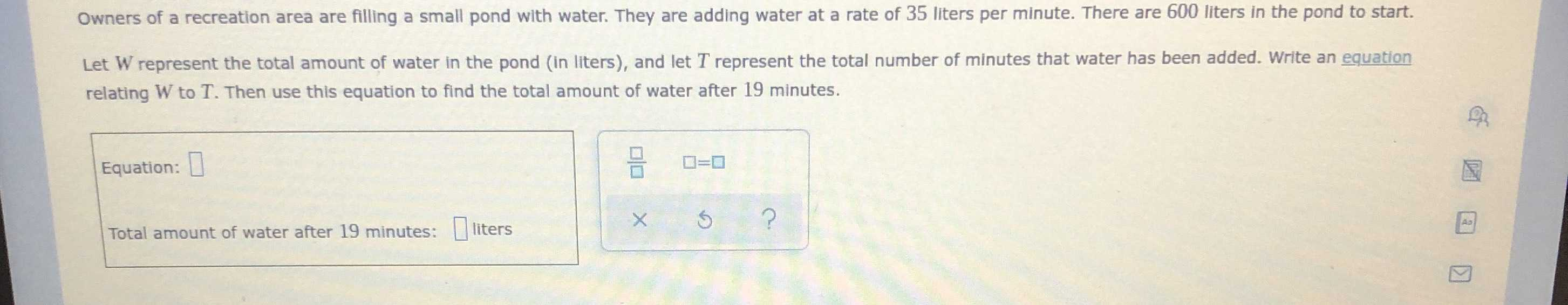### Still have math questions?

Algebra
QuestionOwners of a recreation area are filling a small pond with water. They are adding water at a rate of $$35$$ liters per minute. There are $$600$$ liters in the pond to start. Let $$W$$ represent the total amount of water in the pond (in liters), and let $$T$$ represent the total number of minutes that water has been added. Write an equation relating $$W$$ to $$T$$ . Then use this equation to find the total amount of water after $$19$$ minutes.

Equation: $$\square$$

Total amount of water after $$19$$ minutes: $$\square$$ liters